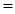Chapter 5, Problem 108SCQ

Chapter
Section
Textbook Problem

Water can be decomposed to its elements, H2 and O2, using electrical energy or in a series of chemical reactions. The following sequence of reactions is one possibility:CaBr2(s) + H2O(g) → CaO(s) + 2 HBr(g)Hg(ℓ) + 2 HBr(g) → HgBr2(s) + H2(g)HgBr2(s) + CaO(s) → HgO(s) + CaBr2(s)HgO(s) → Hg(ℓ) + ½ O2(g)(a) Show that the net result of this series of reactions is the decomposition of water to its elements.(b) If you use 1000. kg of water, what mass of H2 can be produced?(c) Calculate the value of ΔrH° for each step in the series. Are the reactions predicted to be exo- or endothermic?ΔfH° [CaBr2(s)] = –683.2 kJ/molΔfH° [HgBr2(s)] = –169.5 kJ/mol(d) Comment on the commercial feasibility of using this series of reactions to produce H2(g) from water.

(a)

Interpretation Introduction

Interpretation:

To show the net result is the decomposition of water

Concept Introduction:

Heat energy required to raise the temperature of 1g of substance by 1K.Energy gained or lost can be calculated using the below equation.

q=C×m×ΔT

Where, q= energy gained or lost for a given mass of substance (m), C =specific heat capacity, ΔT= change in temperature.

The standard molar enthalpy of formation is the enthalpy change ΔfH0 is the enthalpy change for the formation of 1mol of a compound directly from its component elements in their standard states. And is given by

ΔrH0ΣnΔfH0(products)-ΣnΔfH0(reactants)

Explanation

To show the net result is the decomposition of water.

Equation 1-2=A

A=CaBr2+H2O+HgCaO+HgBr2+H2

Equation A-3=

(b)

Interpretation Introduction

Interpretation:

If 1000Kg of water is used then identify the mass of H2 produced

Concept Introduction:

Heat energy required to raise the temperature of 1g of substance by 1K.Energy gained or lost can be calculated using the below equation.

q=C×m×ΔT

Where, q= energy gained or lost for a given mass of substance (m), C =specific heat capacity,ΔT= change in temperature.

The standard molar enthalpy of formation is the enthalpy change ΔfH0 is the enthalpy change for the formation of 1mol of a compound directly from its component elements in their standard states. And is given by

ΔrH0ΣnΔfH0(products)-ΣnΔfH0(reactants)

(c)

Interpretation Introduction

Interpretation:

The enthalpy of reaction has to be calculated.

Concept Introduction:

Heat energy required to raise the temperature of 1g of substance by 1K.Energy gained or lost can be calculated using the below equation.

q=C×m×ΔT

Where, q= energy gained or lost for a given mass of substance (m), C =specific heat capacity,ΔT= change in temperature.

The standard molar enthalpy of formation is the enthalpy change ΔfH0 is the enthalpy change for the formation of 1mol of a compound directly from its component elements in their standard states. And is given by

ΔrH0ΣnΔfH0(products)-ΣnΔfH0(reactants)

(d)

Interpretation Introduction

Interpretation:

Comment on the feasibility of using such a series of reaction to produce H2

Concept Introduction:

Heat energy required to raise the temperature of 1g of substance by 1K.Energy gained or lost can be calculated using the below equation.

q=C×m×ΔT

Where, q= energy gained or lost for a given mass of substance (m), C =specific heat capacity,ΔT= change in temperature.

The standard molar enthalpy of formation is the enthalpy change ΔfH0 is the enthalpy change for the formation of 1mol a compound directly from its component elements in their standard states. And is given by

ΔrH0ΣnΔfH0(products)-ΣnΔfH0(reactants)

Still sussing out bartleby?

Check out a sample textbook solution.

See a sample solution

The Solution to Your Study Problems

Bartleby provides explanations to thousands of textbook problems written by our experts, many with advanced degrees!

Get Started

Find more solutions based on key concepts